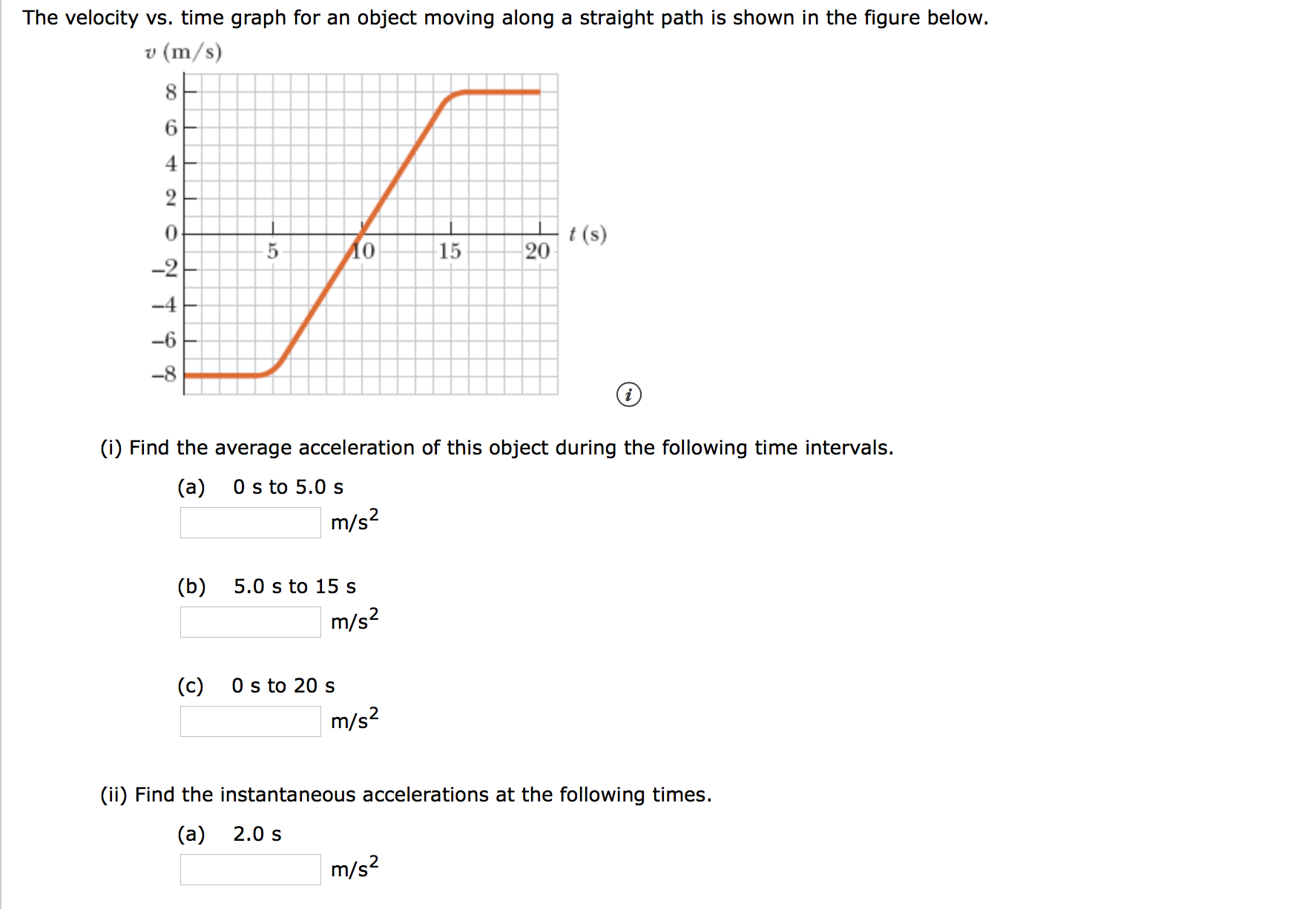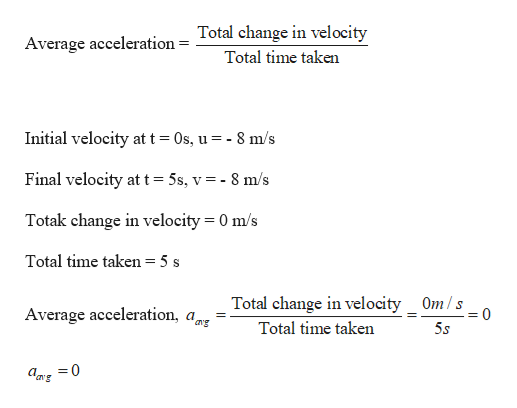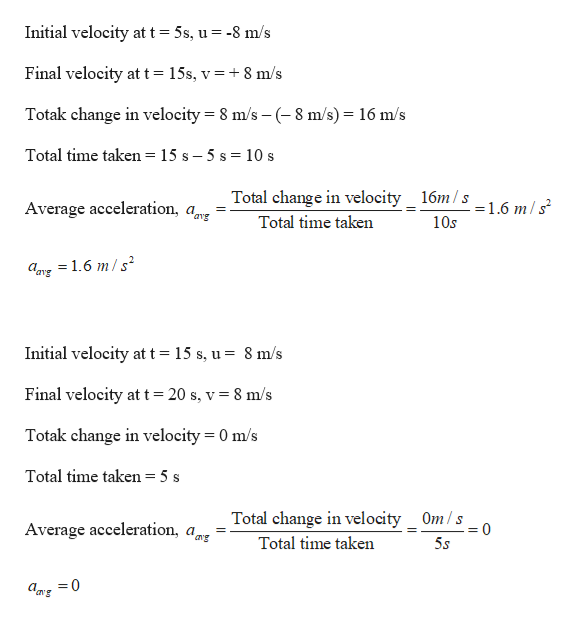# The velocity vs. time graph for an object moving along a straight path is shown in the figure below.(m/s)642t(s)201510-2-4-8(i) Find the average acceleration of this object during the following time intervals.0 s to 5.0 s(a)m/s25.0 s to 15 s(b)m/s2(c)0 s to 20 sm/s2(ii) Find the instantaneous accelerations at the following times.2.0 s(a)m/s2 10 s(b)m/s218 s(c)m/s2

Question
532 viewshelp_outlineImage TranscriptioncloseThe velocity vs. time graph for an object moving along a straight path is shown in the figure below. (m/s) 6 4 2 t(s) 20 15 10 -2 -4 -8 (i) Find the average acceleration of this object during the following time intervals. 0 s to 5.0 s (a) m/s2 5.0 s to 15 s (b) m/s2 (c) 0 s to 20 s m/s2 (ii) Find the instantaneous accelerations at the following times. 2.0 s (a) m/s2 fullscreenhelp_outlineImage Transcriptionclose10 s (b) m/s2 18 s (c) m/s2 fullscreen
check_circle

Step 1

Given:

Step 2

(i) Calculating the average acceleration of the object for part (a):help_outlineImage TranscriptioncloseAverage acceleration = Total change in velocity Total time taken Initial velocity at t 0s, u = - 8 m/s Final velocity at t = 5s, v = - 8 m/s Totak change in velocity = 0 m/s - Total time taken = 5 s Total change in velocity Om/s Average acceleration, a, 0 Total time taken 5.s amg =0 fullscreen
Step 3

Calculating the average acceler...help_outlineImage TranscriptioncloseInitial velocity at t 5s, u = -8 m/s Final velocity at t = 15s, v = + 8 m/s Totak change in velocity 8 m/s (8 m/s) 16 m/s Total time taken = 15 s - 5 s = 10 s Total change in velocity_ 16m/s _16 m/s Average acceleration, ar ang Total time taken 10s aay 1.6 m/s2 Initial velocity at t = 15 s, u = 8 m/s Final velocity at t = 20 s, v = 8 m/s Totak change in velocity 0 m/s Total time taken = 5 s Total change in velocity Om/s Average acceleration, 0 ang Total time taken 5.s an's fullscreen

### Want to see the full answer?

See Solution

#### Want to see this answer and more?

Solutions are written by subject experts who are available 24/7. Questions are typically answered within 1 hour.*

See Solution
*Response times may vary by subject and question.
Tagged in
SciencePhysics

### Kinematics